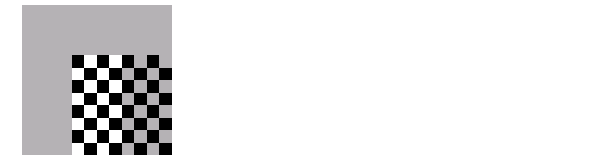Image Processing Toolbox User's GuidePerforming the Spatial Transformation

Once you specify the transformation in a `TFORM` struct, you can perform the transformation by calling `imtransform`. The `imtransform` function performs the specified transformation on the coordinates of the input image and creates an output image.

The translation example called `imtransform` to perform the transformation, passing it the image to be transformed and the `TFORM` structure. `imtransform` returns the transformed image.

• ```cb_trans = imtransform(cb, tform_translate);
```

`imtransform` supports several optional input parameters that you can use to control various aspects of the transformation so as the size of the output image and the fill value used. To see an example of using the `XData` and `YData` input parameters, see Example: Performing Image Registration. For more information about specifying fill values, see Specifying Fill Values.

Specifying Fill Values

When you perform a transformation, there are often pixels in the output image that are not part of the original input image. These pixels must be assigned some value, called a fill value. By default, `imtransform` sets these pixels to zero and they are displayed as black. Using the `FillValues` parameter with the `imtransform` function, you can specify a different color.

Grayscale Images.   If the image being transformed is a grayscale image, you must specify a scalar value that specifies a shade of gray.

For example, in Step 5: View the Output Image, where the example displays the translated checkerboard image in relation to the original coordinate space, you can specify a fill value that matches the color of the gray squares, rather than the default black color.

• ``````cb_fill = imtransform(cb, tform_translate,...
````                  'XData', [1 (size(cb,2)+`xform(3,1)```)],...
````                  'YData', [1 (size(cb,1)+`xform(3,2)```)],...
``````                  'FillValues', .7 );
``````figure, imshow(cb_fill)
``````

Translated Image with Gray Fill Value

RGB Images.   If the image being transformed is an RGB image, you can use either a scalar value or a 1-by-3 vector. If you specify a scalar, `imtransform` uses that shade of gray for each plane of the RGB image. If you specify a 1-by-3 vector, `imtransform` interprets the values as an RGB color value.

For example, this code translates an RGB image, specifying an RGB color value as the fill value. The example specifies one of the light green values in the image as the fill value.

• ``````rgb = imread('onion.png');
```xform = [ 1 0 0
0 1 0
40 40 1 ]
tform_translate = maketform('affine',xform);
cb_rgb = imtransform(rgb, tform_translate,...
'XData', [1 (size(rgb,2)+xform(3,1))],...
'YData', [1 (size(rgb,1)+xform(3,2))],...
'FillValues', [187;192;57]);
figure, imshow(cb_rgb)```

Translated RGB Image with Color Fill Value

If you are transforming multiple RGB images, you can specify different fill values for each RGB image. For example, if you want to transform a series of 10 RGB images, a 4-D array with dimensions 200-by-200-by-3-by-10, you have several options. You can specify a single scalar value and use a grayscale fill value for each RGB image. You can also specify a 1-by-3 vector to use a single color value for all the RGB images in the series. To use a different color fill value for each RGB image in the series, specify a 3-by-10 array containing RGB color values.Creating TFORM Structures Performing N-Dimensional Spatial Transformations